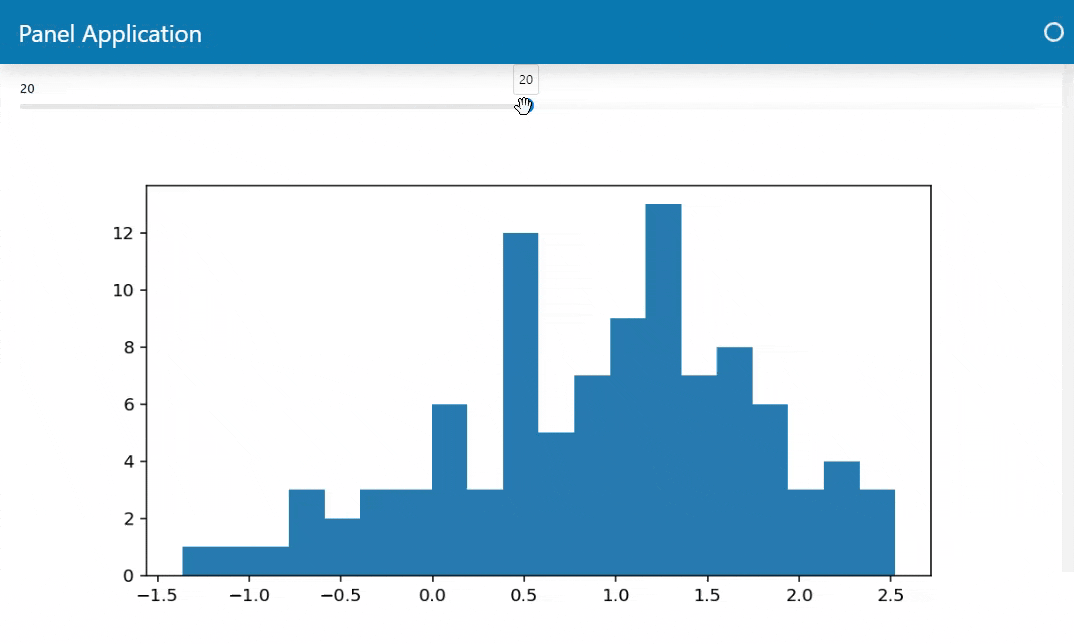# Improve the performance with Caching#

One of the key concepts in Streamlit is caching.

In Streamlit

• your script is run once when a user visits the page.

• your script is rerun top to bottom on user interactions.

Thus with Streamlit you must use caching to make the user experience nice and fast.

In Panel

• your script is run once when a user visits the page.

• only specific, bound functions are rerun on user interactions.

Thus with Panel you may use caching to to make the user experience nice and fast.

In Panel you use `pn.cache` to speed up your apps. Check out the Cache How-To Guides for more details.

## Migration Steps#

To migrate

• replace `st.cache_data` and `st.cache_resource` with `pn.cache` on long running

• bound functions

## Example#

### Cache Example#

#### Streamlit Cache Example#

```from time import sleep

import numpy as np
import streamlit as st
from matplotlib.figure import Figure

@st.cache_data
def get_data():
print("get_data func")
sleep(1.0)
return np.random.normal(1, 1, size=100)

@st.cache_data(hash_funcs={Figure: lambda _: None})
def plot(data, bins):
print("plot func", bins)
sleep(2)
fig = Figure(figsize=(8,4))
ax = fig.subplots()
ax.hist(data, bins=bins)
return fig

data = get_data()
bins = st.slider(value=20, min_value=10, max_value=30, step=1, label="Bins")
st.pyplot(plot(data, bins))
```

I’ve added `sleep` statements to make the functions more expensive.

#### Panel Cache Example#

```from time import sleep

import numpy as np
import panel as pn
from matplotlib.figure import Figure

@pn.cache
def get_data():
print("get_data func")
sleep(1.0)
return np.random.normal(1, 1, size=100)

@pn.cache
def plot(data, bins):
print("plot func", bins)
sleep(2)
fig = Figure(figsize=(8,4))
ax = fig.subplots()
ax.hist(data, bins=bins)
return fig

pn.extension(sizing_mode="stretch_width", template="bootstrap")

data = get_data()
bins = pn.widgets.IntSlider(value=20, start=10, end=30, step=1)
bplot = pn.bind(plot, data, bins)You can also use `pn.cache` as an function. I.e. as
```plot = pn.cache(plot)
Using `pn.cache` as a function can help you keep your business logic (`data` and `plot` function) and your caching logic (when and how to apply caching) separate. This can help you reusable and maintainable code.